# Little Man Tate 1991

MOV, Ogg Webm. IMDb link A nice movie directed by Jodie Foster who plays also the Mother of Fred. There are some arithmetic computations in. For example
• 465*24*60*48 = 32140800
• 3067 is prime.
• For the riddle to solve the system of nonlinear Diophantine equations (a+10b)/(a*b) =3, a+10b+18=b+10a with solution a=4,b=3. A complete solution would also have to establish that there are no solutions for numbers larger than 100 but that is harder to see: here is the computer algebra verification for 3 digits or 4 digit numbers.
Solve[{(a+10b+100c)/(a*b*c)==3,a+10b+100c+18==c+10b+100a},{a,b,c}]
Solve[{(a+10b+100c+1000d)==3*a*b*c*d,a+10b+100c+1000d+18==d+10c+100b+1000a},{a,b,c,d}]
• In the problem to take the cube root of 3796466. There is an error in the movie as 156^3 = 3796416.
• The riddle with the cube and squares is not very clearly stated but it requires to find a root of the polynomial x^3 + 5x^2-42*x-40 where 5 solves it.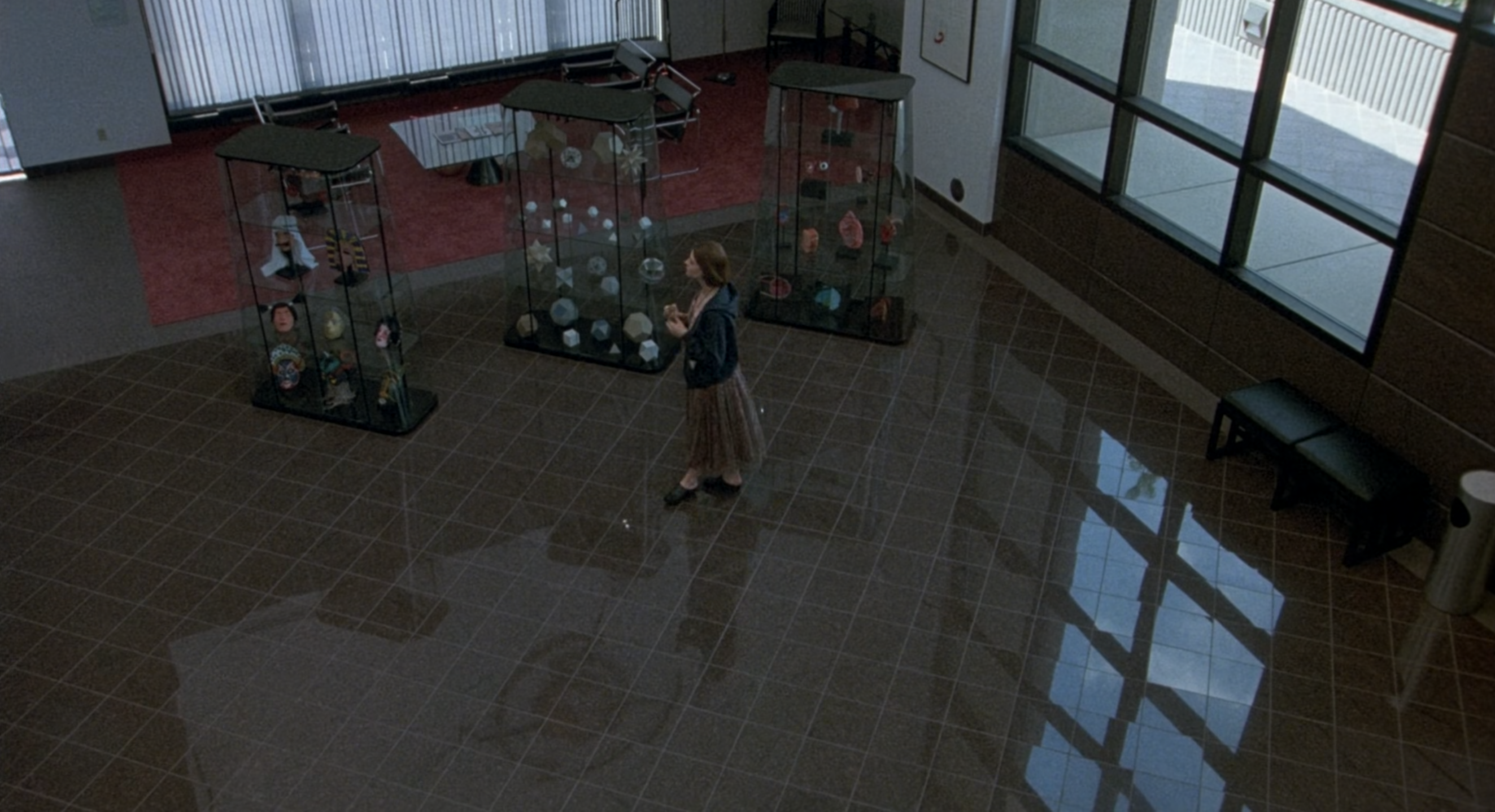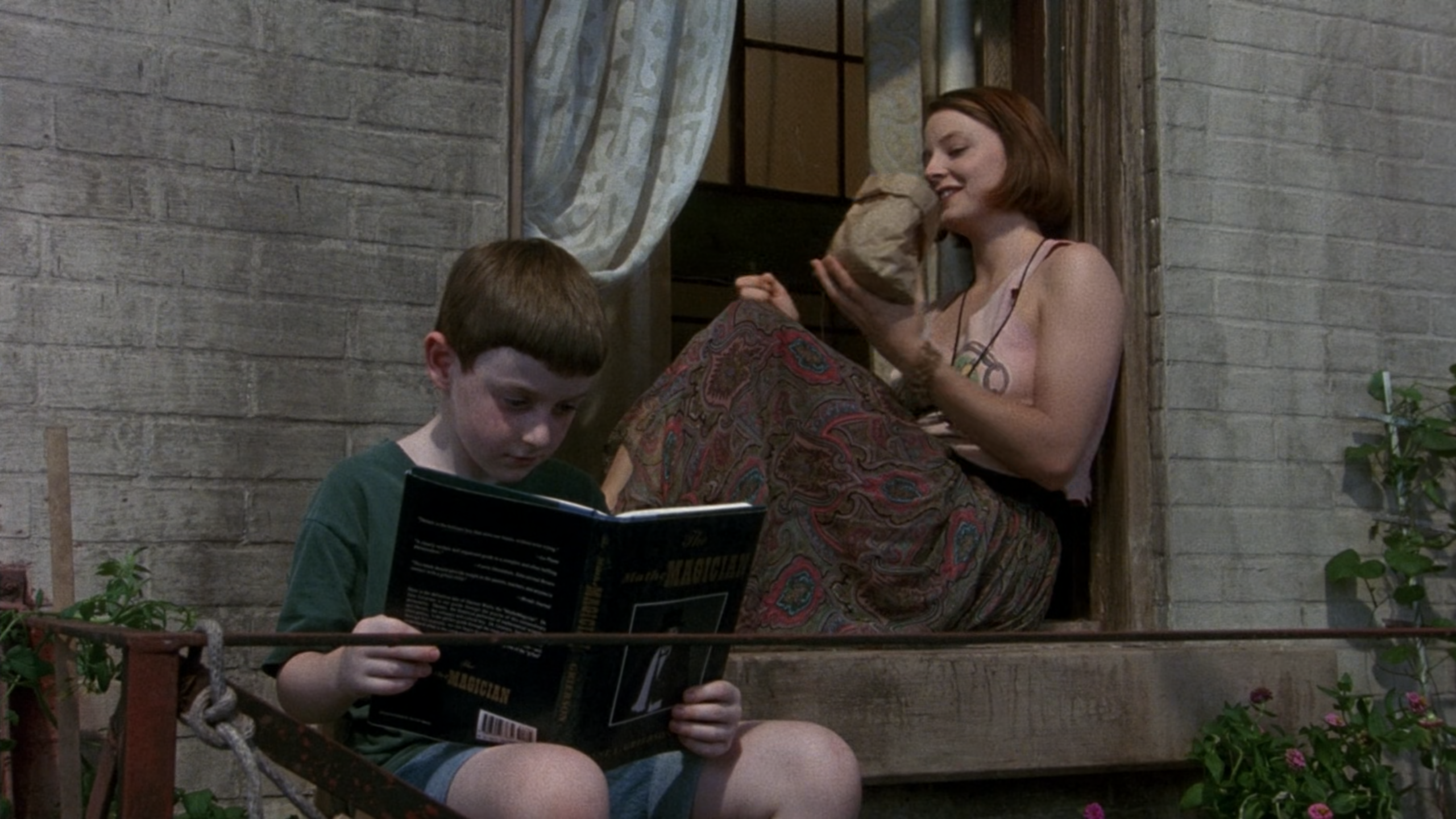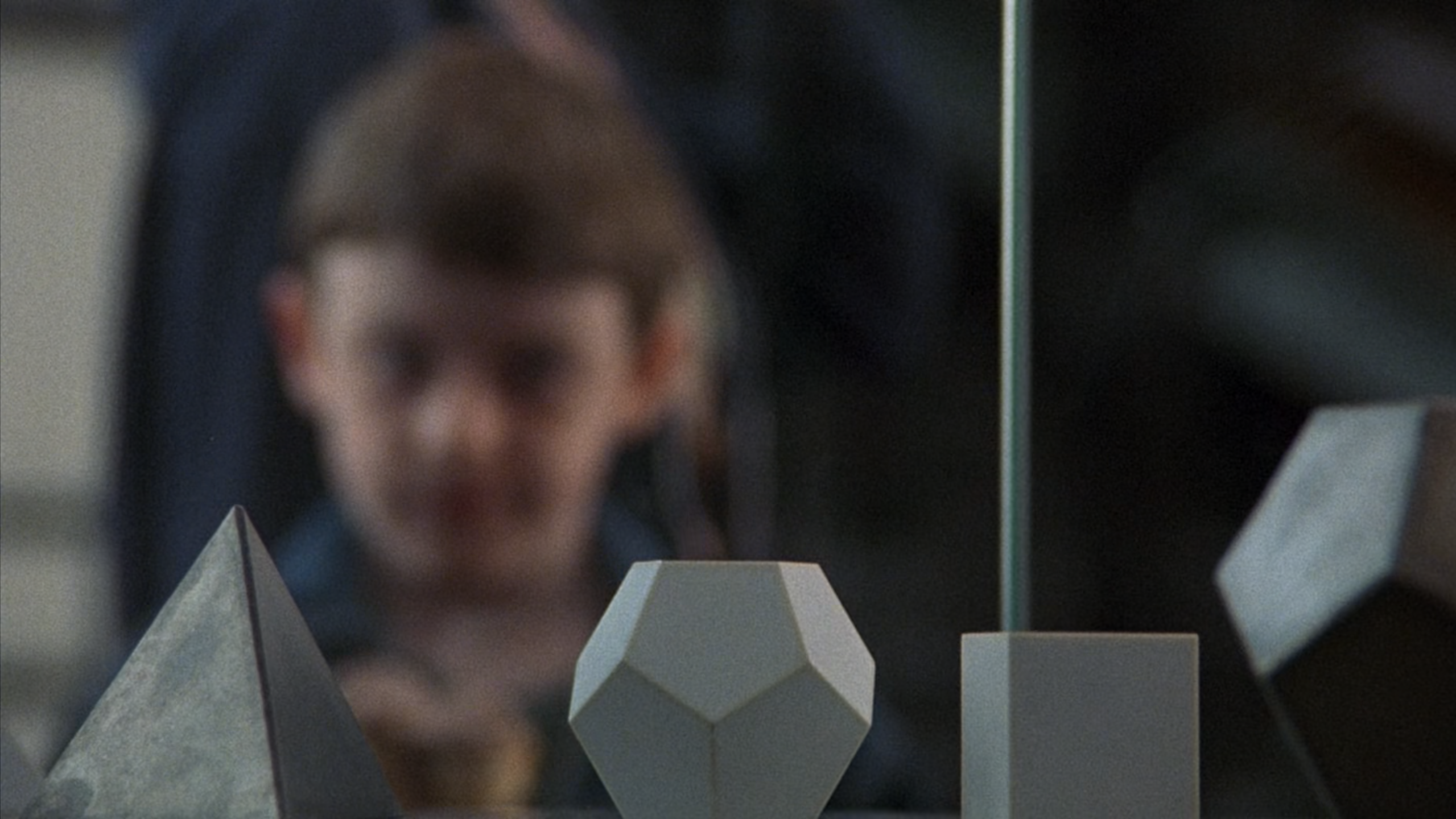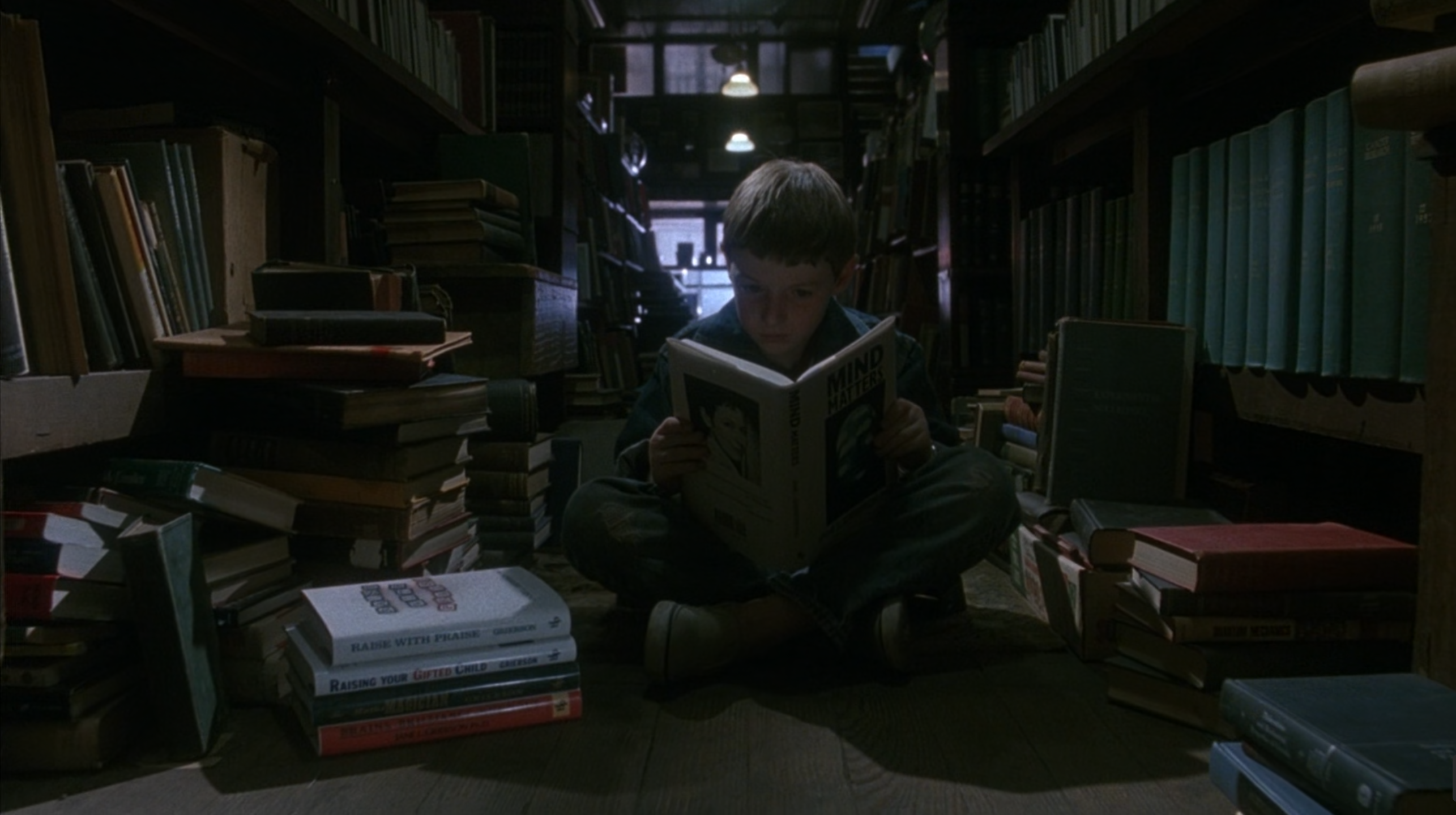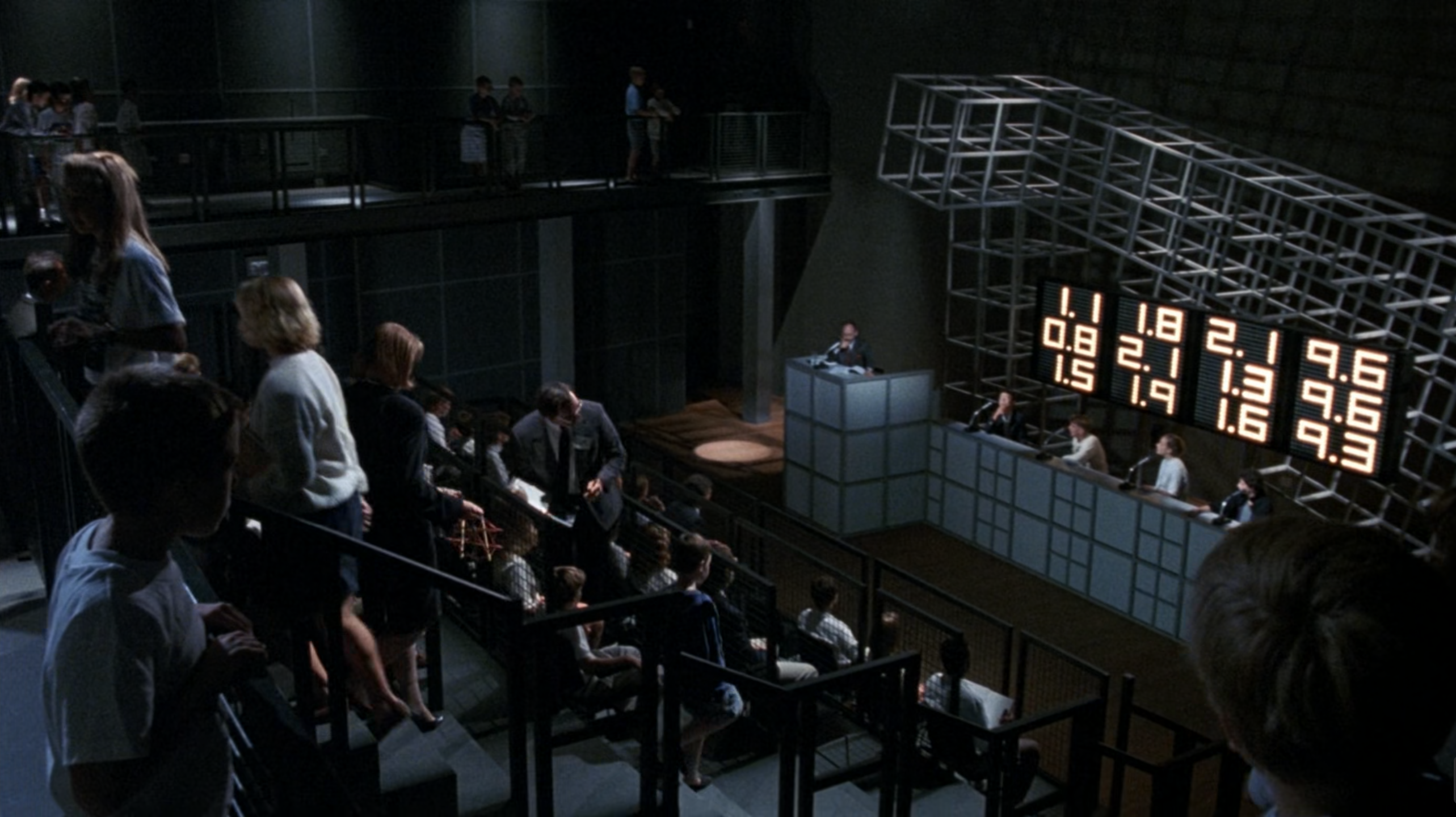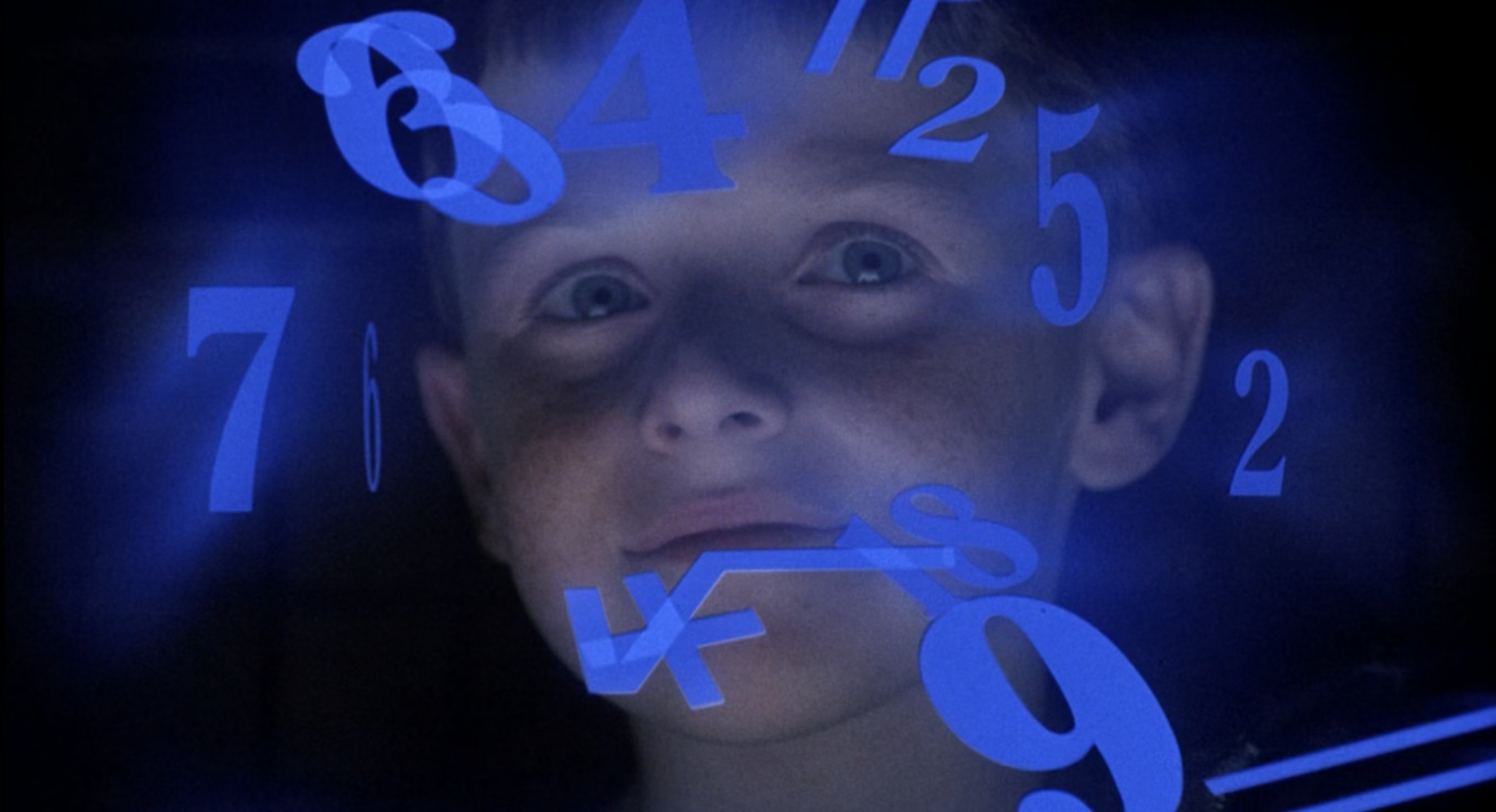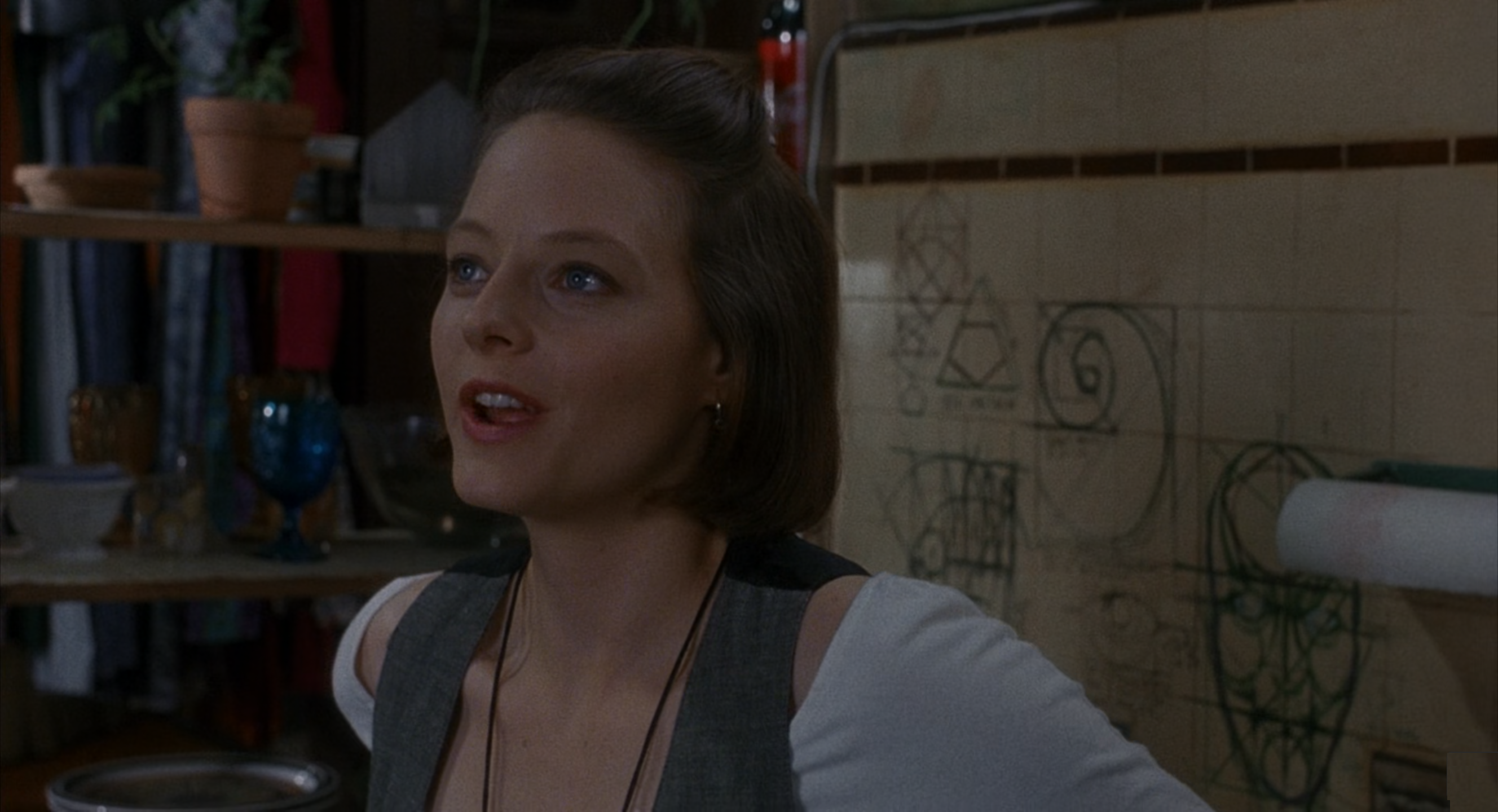Oliver Knill, Posted December 5, 2021,